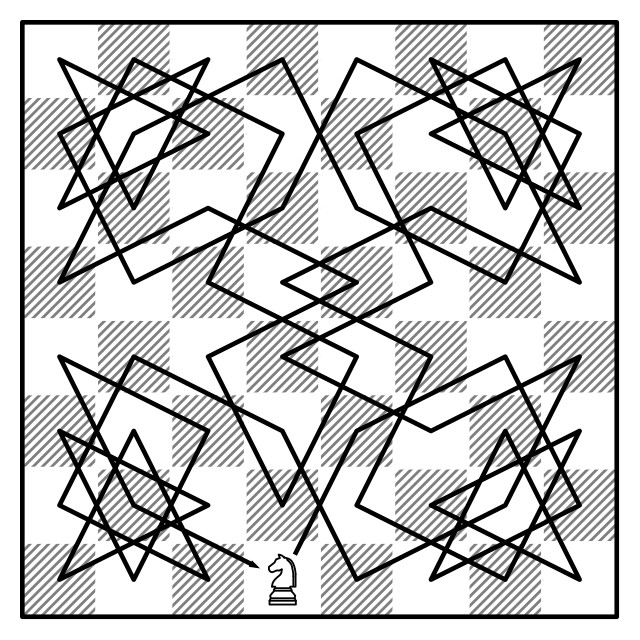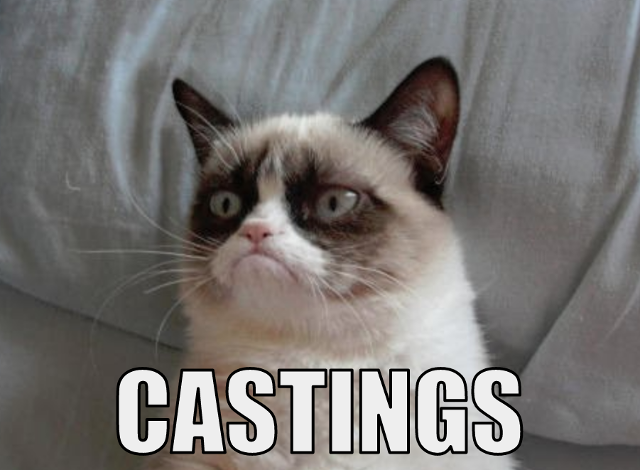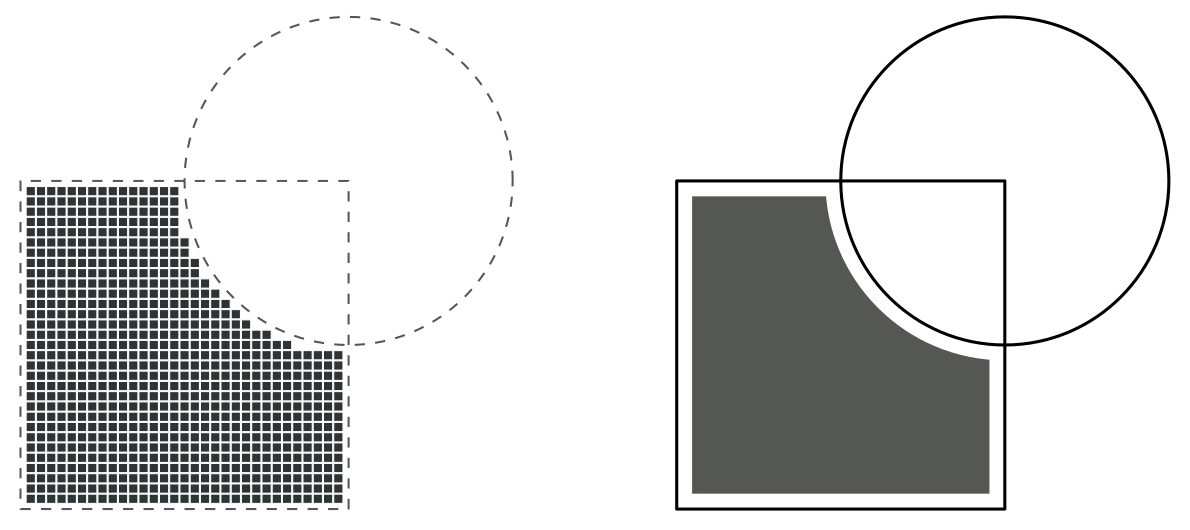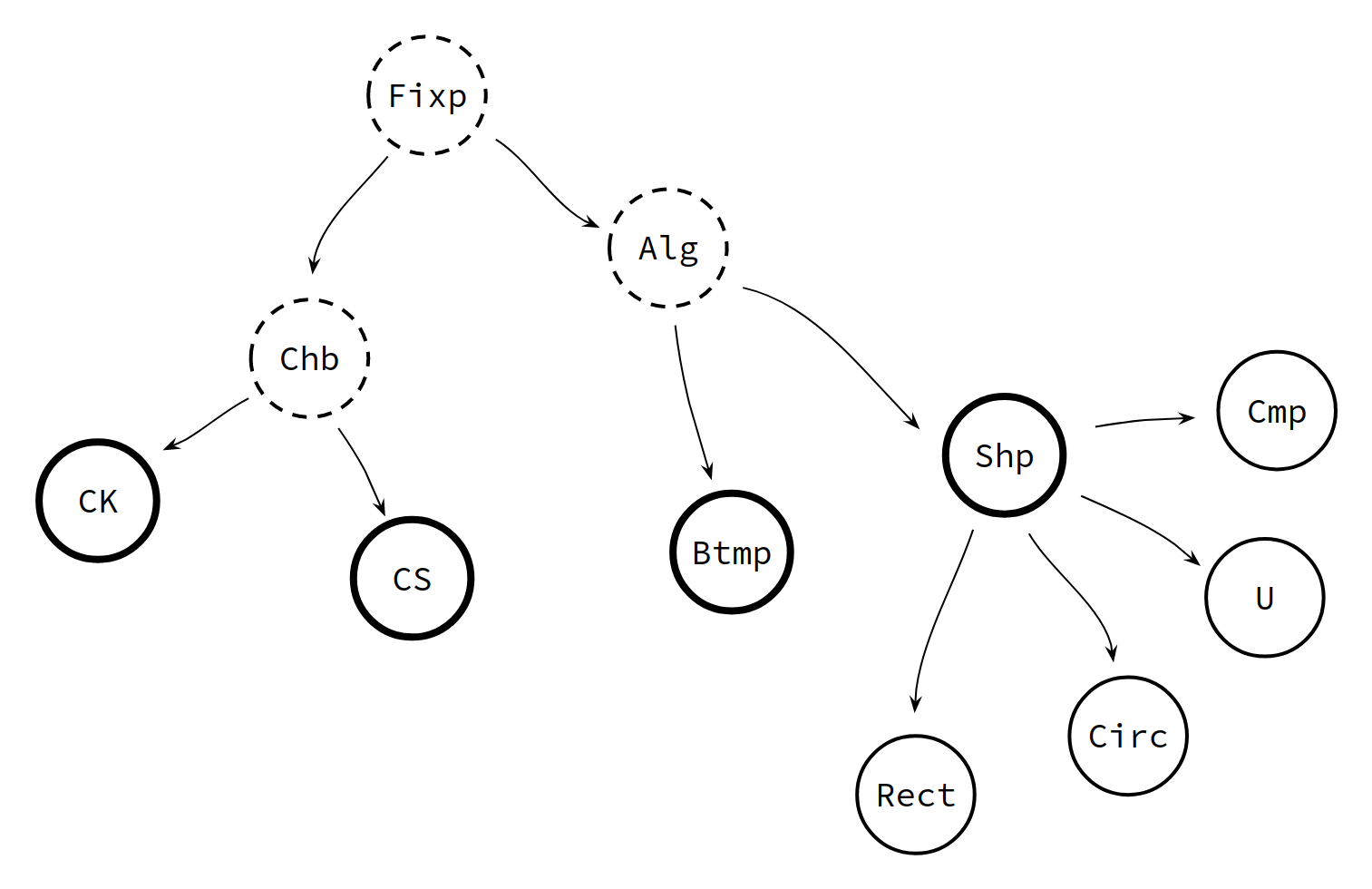Castings in Java are considered a bad practice.

Java’s strong static typing is a powerful compile-time safeguard. It checks that every method receives and returns objects with the features they are supposed to have. It makes sure that everything is well assembled.

Castings open a backdoor in this mechanism by allowing unchecked type transformations between classes involved in an inheritance. Although castings hardly have any impact in performance, they may lead to runtime errors, which in part defeats the very purpose of strong static typing.

It is commonly agreed that a repeated use of castings denotes a bad design. However, there are some cases in which avoiding them is far from obvious. One of these cases is having several subclasses inheriting from a superclass whose methods have argument or return types referring to the subclasses themselves:

``````public class A {
public A method(A other) {...};
}

public class B extends A {
@Override
public B method(B other) {...}; // !!
}
``````

Is it possible to avoid castings here?

# A Tale of Two Castings

Suppose that we have found a really good algorithm to find long chains of elements. It takes elements from a `Set<T>`, and for any two of them, it needs to know if they may be chained. With this information, it efficiently builds long chains of elements. For instance, it may find knight tours in a chessboardor it could create lists of words that change only one letter in each step:

``````PASS
PAST
CAST
COST
MOST
LOST
LOFT
...
``````

The skeleton of our `Chainer` would be something like this:

``````public class Chainer<T> {

final Set<T> items;

public Chainer(final Set<T> items) {
this.items = items;
}

public List<T> longChain() {
// try to build a very long chain
//
// during the process, lots of pairs of elements are taken from items;
// for each pair we need to check whether they may be chained or not
};

public List<T> joiningChain(T a, T b) {
// try to build a chain joining a and b - again, pairs of elements
// are checked for "chainability"
};
}
``````

The type `T` does not provide the logic of chainable elements. So we build a `Chainable` interface to provide this logic:

``````// Chainer.java
public class Chainer<T extends Chainable> { /* ... */ }

// Chainable.java
public interface Chainable {
public boolean mayBeChainedTo(Chainable other);
}

// CKnight.java
public class CKnight implements Chainable {
public boolean mayBeChainedTo(Chainable other) { /* ... */ }
}

// CString.java
public class CString implements Chainable {
public boolean mayBeChainedTo(Chainable other) { /* ... */ }
}
``````

You see, we already have two examples of `Chainable`: chessboard squares and strings. Two squares may be chained if connected by a single knight jump, and two strings may be chained if only one letter differs. The logic itself is not very hard to implement. However, one may perceive a little subtlety with the types.

``````// CKnight.java
public boolean mayBeChainedTo(Chainable other) {
CKnight k = (CKnight)other;
// ...
}
``````

The right thing would be to compare a `CKnight` with another `CKnight`, but the inherited method requires comparing with a `Chainable`. This forces us to begin the comparison with a downcasting.Another possibility would be to provide a `BiPredicate<T,T>` to the `Chainer`

``````public class Chainer<T> {

final Set<T> items;
final BiPredicate<T,T> areChainable;
// ...
}
``````

but this would defeat our aim to encapsulate the behavior in the type itself.

Anoter example, this one a bit more complicated: imagine that we are building an applet that deals with 2-D geometric elements: points, curves, polygons, circles, splines, …

One may conceive different approaches to model 2-D regions. We could think of a numerical approach, where we would model the region using some sort of bitmap, or we could go towards a more analytic approach, keeping track of the atomic components. And perhaps both approaches are useful and can be used simultaneously (one to draw stuff on the screen and the other to perform accurate calculations).All the approaches would need to implement a similar set of algebraic operations including union, intersection, complement and difference. Some of these operations may be computed in terms of others. This way we create the `AlgebraicSet` interface. The interface provides a default implementation for `intersection` and `diff`, calculated using the other two operations (`union` and `complement`).

``````public interface AlgebraicSet {

public AlgebraicSet union(AlgebraicSet other);
public AlgebraicSet complement();

// --------

public default AlgebraicSet intersection(AlgebraicSet other) {
return this.complement().union(other.complement()).complement();
}

public default AlgebraicSet diff(AlgebraicSet other) {
return this.intersection(other.complement());
}

}
``````

Cool!! Let’s now implement `BitMap`.

``````public class BitMap extends AlgebraicSet {

public BitMap(/* ... */) {
// perhaps it could be implemented as some sort of double array of booleans?
}

public BitMap union(AlgebraicSet other) {
BitMap bitmapOther = (BitMap)other;
// bitwise AND
return new BitMap(/* ... */);
}
public BitMap complement() {
// bitwise NOT
return new BitMap(/* ... */);
}

}
``````

Did you notice?And this time it’s slightly worse: there is a downcasting for the argument type much like in `Chainable`, but there’s also a difference in the return type. The initial methods to be implemented were `AlgebraicSet union(AlgebraicSet other)` and `AlgebraicSet complement()`; Java allows tweaking the return type with `BitMap union(AlgebraicSet other)` and `BitMap complement()`, but it’s still ugly nevertheless.

Let’s hope better luck for our symbolic approach in `Shape`:

``````public abstract class Shape implements AlgebraicSet {

// union and complement in terms of certain subclases
public Shape union(AlgebraicSet other) {
Shape symbOther = (Shape)other; // Casting :/
return new Union(this, symbOther);
}
public Shape complement() {
return new Complement(this);
}

// checking whether a region contains a point
// BitMap does not implement it because it's not accurate enough
public abstract boolean contains(double x, double y);

// --------

public static class Union extends Shape {
protected Shape a;
protected Shape b;

public Union(Shape a, Shape b) {
this.a = a;
this.b = b;
}

public boolean contains(double x, double y) {
return a.contains(x, y) || b.contains(x, y);
}

}

public static class Complement extends Shape {
protected Shape a;

public Complement(Shape a) {
this.a = a;
}

public boolean contains(double x, double y) {
return !a.contains(x, y);
}

}

// a couple of basic shapes

public static class Rectangle extends Shape {
protected double x0;
protected double x1;
protected double y0;
protected double y1;

public Rectangle(double x0, double x1, double y0, double y1) {
this.x0 = x0;
this.x1 = x1;
this.y0 = y0;
this.y1 = y1;
}

public boolean contains(double x, double y) {
return x0 <= x && x <= x1 && y0 <= y && y <= y1;
}

}

public static class Circle extends Shape {
protected double xc;
protected double yc;
protected double r;

public Circle(double xc, double yc, double r) {
this.xc = xc;
this.yc = yc;
this.r = r;
}

public boolean contains(double x, double y) {
return (x-xc)*(x-xc) + (y-yc)*(y-yc) <= r*r;
}

}

}
``````

Ok. No problem so far. Let’s check that everything’s peachy.

``````new Rectangle(-1,1,-1,1).union(new Circle(1,1,1)).contains(0,0);
// OK --> true
``````

Great.

``````new Rectangle(-1,1,-1,1).diff(new Circle(1,1,1)).contains(0,0);
// KO --> The method contains(int, int) is undefined for the type AlgebraicSet
``````

What??!! The method `diff` was not implemented in `Shape`, but in `AlgebraicSet`, and therefore its return type is `AlgebraicSet`. Although semantically equivalent, `union` and `diff` work with different types. So each time we use `diff` we have to perform another downcasting

``````((Shape)new Rectangle(-1,1,-1,1).diff(new Circle(1,1,1))).contains(0,0);
// (╯°□°)╯︵ ┻━┻
``````

and this is too much. We need to find a better solution.

# A wannabe type

In both examples, `Chainable` and `AlgebraicSet`, the problem is more or less the same: we want to build certain logic that will be inherited by multiple subclasses, and we need to have the current subclass type in the implementation.

How can we do this? Imagine that we have a type `Base` that will be extended by some type `T`; inside `Base` we want to refer to `T` so… why not? We introduce `T` as a type parameter.

``````class Base<T> // Base will eventually become T
``````

Now we may use `T` to define argument and return types. But, on the other hand, `T` cannot be anything, it has to extend `Base`.

``````class Base<T extends Base>
``````

But `Base` is no longer a raw type; it needs to be told about `T`.

``````class Base<T extends Base<T>>
``````

What’s going on here? Is this a circular definition? Nope, it’s perfect Java syntax. You can impose type predicates on the parameters. If you find some `T` satistying the predicate, you’ll be able to instantiate a `Base<T>`. If the predicate is impossible to fulfill, then you won’t be able to instantiate a `Base<T>`, although everything will compile fine.

Suppose that `Derived` is to extend `Base`

``````class Derived extends Base<Derived>
``````

Look, it works!! `Base<Derived>` is ok because `Derived extends Base<Derived>` satisfies the condition `T extends Base<T>`. And now `Derived` inherits all the logic in `Base` with all the `T` replaced by `Derived`. Think of `Base<T>` as being in mid-metamorphosis towards `T`: that’s why `Derived` extends `Base<Derived>`, because `Base<Derived>` was already prepared to be transformed in `Derived`.

How does this approach translate in our previous examples?

``````// Chainable.java
public interface Chainable<C extends Chainable<C>> {
public boolean mayBeChainedTo(C other);
}

// CKnight.java
public class CKnight implements Chainable<CKnight> {
public boolean mayBeChainedTo(CKnight other) { /* ... */ };
}

// CString.java
public class CString implements Chainable<CString> {
public boolean mayBeChainedTo(CString other) { /* ... */ };
}

// Chainer.java
public class Chainer<C extends Chainable<C>> {

final Set<C> items;

public Chainer(final Set<C> items) {
this.items = items;
}

public List<C> longChain() { /* ... */ };

public List<C> joiningChain(C a, C b) { /* ... */ };

}
``````

Did you see? No castings!

``````// AlgebraicSet.java
public interface AlgebraicSet<A extends AlgebraicSet<A>> {

public A union(A other);
public A complement();

// --------

public default A intersection(A other) {
return this.complement().union(other.complement()).complement();
}

public default A diff(A other) {
return this.intersection(other.complement());
}

}

// BitMap.java
public class BitMap implements AlgebraicSet<BitMap> {

// ...

public BitMap union(BitMap other) {
// bitwise AND
return new BitMap(/* ... */);
}
public BitMap complement() {
// bitwise NOT
return new BitMap(/* ... */);
}

}

// Shape.java
public abstract class Shape implements AlgebraicSet<Shape> {

public Shape union(Shape other) {
return new Union(this, other);
}
public Shape complement() {
return new Complement(this);
}

public abstract boolean contains(double x, double y);

// ...

}
``````

Fabulous! Our types match perfectly!

``````new Rectangle(-1,1,-1,1).union(new Circle(1,1,1)).contains(0,0); // OK
new Rectangle(-1,1,-1,1).diff(new Circle(1,1,1)).contains(0,0);  // OK
``````

Strange? Witchcraft? Well, you’d be surprised to know that this technique is already being used in the core of Java: the keyword `enum`

``````public enum Suit {HEART, DIAMOND, CLUB, SPADE};
``````

is indeed syntactic sugar of a class `Enum` that uses this “recursive definition”

``````class Enum<E extends Enum<E>> {
...
}
``````
``````class Suit extends Enum<Suit> {
public static final Suit HEART;
public static final Suit DIAMOND;
public static final Suit CLUB;

// ...
}
``````

This makes sense because all our `enum` have common properties but we want each subclass to be compared to members of the same subclass, much like with the `Chainable` elements. Yes, sometimes you have to reason in terms of your subclass types.

# Polymorphic Fixpoint

We’re very happy with our trick and ready to move on. Let’s add another common set operation to `AlgebraicSet`: the symmetric difference.

``````public default A symDiff(A other) {
return this.diff(other).union(other.diff(this));
}
// The method diff(A) in the type AlgebraicSet<A>
// is not applicable for the arguments (AlgebraicSet<A>)
``````

Yikes!! Since this method is implemented in the parent class, `this` refers to the parent class, and not to the child class. But we need `this` to be the child class. What can we do?

``````// Base.java
abstract class Base<T extends Base<T>> {
abstract public T getChild();
}

// Derived.java
class Derived extends Base<Derived> {
public Derived getChild() { return this; }
}
``````

Looks good! To formalize this process we’ll create a `Fixpoint` interface that will be implemented by all the classes using this polymorphic fixpoint trick:

``````public interface Fixpoint<X extends Fixpoint<X>> {
public X fixpoint();
}
``````

Let’s introduce `Fixpoint` in `Chainable`

``````// Chainable.java
public interface Chainable<C extends Chainable<C>> extends Fixpoint<C>

// CKnight.java
public CKnight fixpoint() { return this; }

// CString.java
public CString fixpoint() { return this; }
``````

… and in `AlgebraicSet`:

``````// AlgebraicSet.java
public interface AlgebraicSet<A extends AlgebraicSet<A>> extends Fixpoint<A> {

public A union(A other);
public A complement();

// --------

public default A intersection(A other) {
return fixpoint().complement().union(other.complement()).complement();
}

public default A diff(A other) {
return fixpoint().intersection(other.complement());
}

public default A symDiff(A other) {
return fixpoint().diff(other).union(other.diff(fixpoint()));
}

}

// BitMap.java
public BitMap fixpoint() { return this; }

// Shape.java
public Shape fixpoint() { return this; }
``````

Did you see? In `AlgebraicSet` we are now using `fixpoint()`, of type `A`, instead of `this`, of type `AlgebraicSet<A>`. Same happens with `Chainable`.

Why “fixpoint”? Because we’re asking each instance to “evolve” to its “more developed version”, but in the final subclases this evolution is the identity, and thus the `return this;`. And why “polymorphic”? Well, becuase this “more developed version” takes lots of different forms indeed.

Altogether, we have built the following hierarchy of classes/interfaces:The dashed objects incorporate the polymorphic fixpoint trick in their type definition and cannot be instantiated until the thick objects implement `fixpoint() { return this; }` and no longer receive a type parameter. From this moment on, type inheritance is completely standard.

Now we can let our imagination fly and come up with all kinds of fancy stuff, like for example a fluent logger able to write the status of an object at any time

``````public interface Logger<L extends Logger<L>> extends Fixpoint<L> {

default public L log() {
System.out.println(repr());
return fixpoint();
}

public String repr();

}

// BitMap.java
public class BitMap implements AlgebraicSet<BitMap>, Logger<BitMap> {
// ...
public String repr() { /* ... */ }
}

// some other file
new BitMap(/* ... */).log().union(new BitMap(/* ... */));
``````

# Wrapping up

To sum up: the polymorphic fixpoint trick is very useful to avoid castings in situations where a behavior is to be extended by several different implementations but needs to refer to the implementations themselves.

Updated: